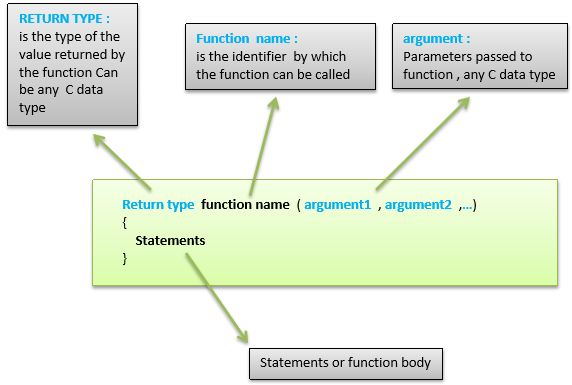# Arduino - Functions

Functions allow structuring the programs in segments of code to perform individual tasks. The typical case for creating a function is when one needs to perform the same action multiple times in a program.
Standardizing code fragments into functions has several advantages −
• Functions help the programmer stay organized. Often this helps to conceptualize the program.
• Functions codify one action in one place so that the function only has to be thought about and debugged once.
• This also reduces chances for errors in modification, if the code needs to be changed.
• Functions make the whole sketch smaller and more compact because sections of code are reused many times.
• They make it easier to reuse code in other programs by making it modular, and using functions often makes the code more readable.
There are two required functions in an Arduino sketch or a program i.e. setup () and loop(). Other functions must be created outside the brackets of these two functions.
The most common syntax to define a function is −## Function Declaration

A function is declared outside any other functions, above or below the loop function.
We can declare the function in two different ways −
The first way is just writing the part of the function called a function prototype above the loop function, which consists of −
• Function return type
• Function name
• Function argument type, no need to write the argument name
Function prototype must be followed by a semicolon ( ; ).
The following example shows the demonstration of the function declaration using the first method.

### Example

`int sum_func (int x, int y) // function declaration {   int z = 0;   z = x+y ;   return z; // return the value}void setup () {   Statements // group of statements}Void loop () {   int result = 0 ;   result = Sum_func (5,6) ; // function call}`
The second part, which is called the function definition or declaration, must be declared below the loop function, which consists of −
• Function return type
• Function name
• Function argument type, here you must add the argument name
• The function body (statements inside the function executing when the function is called)
The following example demonstrates the declaration of function using the second method.

### Example

`int sum_func (int , int ) ; // function prototypevoid setup () {   Statements // group of statements}Void loop () {   int result = 0 ;   result = Sum_func (5,6) ; // function call}int sum_func (int x, int y) // function declaration {   int z = 0;   z = x+y ;   return z; // return the value}`
The second method just declares the function above the loop function.

arduino projects for beginners
arduino projects with code
arduino projects for kids
arduino projects book
arduino projects for engineering students
arduino projects ideas
arduino projects for dummies
arduino projects for beginners pdf
arduino projects amazon
arduino projects art
arduino projects app
arduino projects agriculture
arduino projects and codes
arduino projects alarm system
arduino projects automotive
the arduino projects book
arduino projects beginner
arduino projects book code
arduino projects beginner kit
arduino projects based on agriculture
arduino projects biomedical
raspberry pi 3 b arduino projects
arduino projects code
arduino projects cool
arduino projects c++
arduino projects car
arduino projects covid
arduino projects covid 19
arduino projects circuit digest
arduino projects course
arduino cc projects
arduino c programming projects
arduino projects diy
arduino projects drone
arduino projects diagram
arduino projects distance sensor
arduino projects digital clock
arduino projects door lock
arduino projects dc motor control
d arduino
arduino projects easy
arduino projects examples
arduino projects electronics hub
arduino projects engineering
arduino projects easy to make
arduino projects ebook
arduino projects explained
arduino projects elevator
arduino e bike projects
arduino projects for high school students
arduino projects for beginners with code
f() arduino
arduino projects github
arduino projects games
arduino projects garden
arduino projects greenhouse
arduino projects gps tracker
arduino projects gsm module
arduino projects guitar
arduino projects gyroscope
arduino project hub
arduino projects home automation